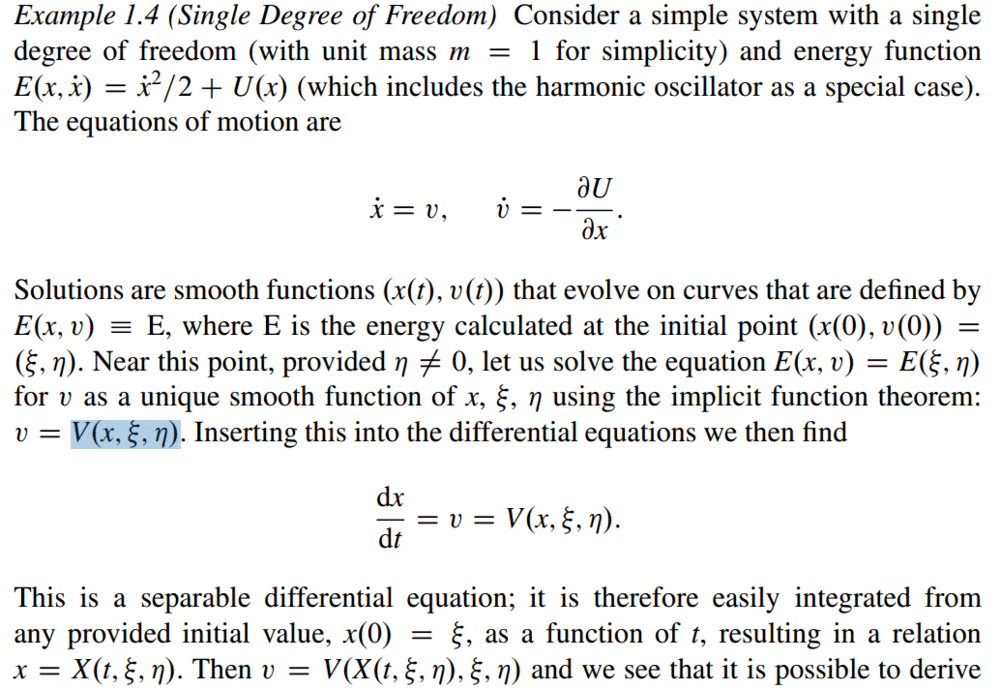# Question about this Separable ODE statement in a book

Summary:
A question to a statement in a book.
Greetings,

I have a question to the following section of the book https://www.springer.com/gp/book/9783319163741:I understand that the equation is separable, since I can just write
$$\int_{x_0}^{x} \frac {1}{V(x', \xi, \eta)}dx' =\int_{0}^{t}dt' .$$
However, without knowing the exact shape of the function ##V##, how can I know that I can bring the resulting formula into the shape ##x=X(t, \xi, \eta)##? Am I missing something or is the author a bit too imprecise here?

Best,
SL

Last edited:

## Answers and Replies

anuttarasammyak
Gold Member
From your equation, say
$$\int^x_C \frac{1}{V}dx'=G(x,\xi,\eta),$$
$$G(x,\xi,\eta)-G(x_0,\xi,\eta)=t-t_0$$
$$x=G^{-1}(t-t_0+G(x_0,\xi,\eta))=X(t,\xi,\eta,t_0,x_0)$$
where ##G^{-1}## is a inverse function of G as for x with ##\xi,\eta## and ##x_0##,##t_0=0## given.

•SchroedingersLion
Thanks!

What is the prerequisite for the integral function having an inverse?

anuttarasammyak
Gold Member
Integral should be monotonous and not diverge so V does not change sign in the region.

wrobel
Science Advisor
I find the formula ##v=V(x,\xi,\eta)## strange. I would write ##E(x,v)=h(=const)##. Assume that for some ##x_0,v_0,\quad E(x_0,v_0)=h## we have ##\frac{\partial E}{\partial v}(x_0,v_0)\ne 0## then for some small enough ##|x-x_0|## there is a unique function ##v=V(x)## such that ##E(x, V(x))\equiv h,\quad v_0=V(x_0).##

Last edited:
Integral should be monotonous and not diverge so V does not change sign in the region.
How can you tell?

I find the formula ##v=V(x,\xi,\eta)## strange. I would write ##E(x,v)=h(=const)##. Assume that for some ##x_0,v_0,\quad E(x_0,v_0)=h## we have ##\frac{\partial E}{\partial v}(x_0,v_0)\ne 0## then for some small enough ##|x-x_0|## there is a unique function ##v=V(x)## such that ##E(x, V(x))\equiv h,\quad v_0=V(x_0).##
But this is the same as the text says, your ##(x_0, v_0)## corresponds to its ##(\xi, \eta)##.

wrobel
Science Advisor
But this is the same as the text says,
I have reread the text and yes, it is practically the same. Regarding your initial question I think that these formulas should be taken formally, in concrete examples everything is as usual clear. You have just been shown that the system is solvable in quadratures. This integral does not actually play a crucial role. Phase diagrams play

Thanks for the drawing, I understand!